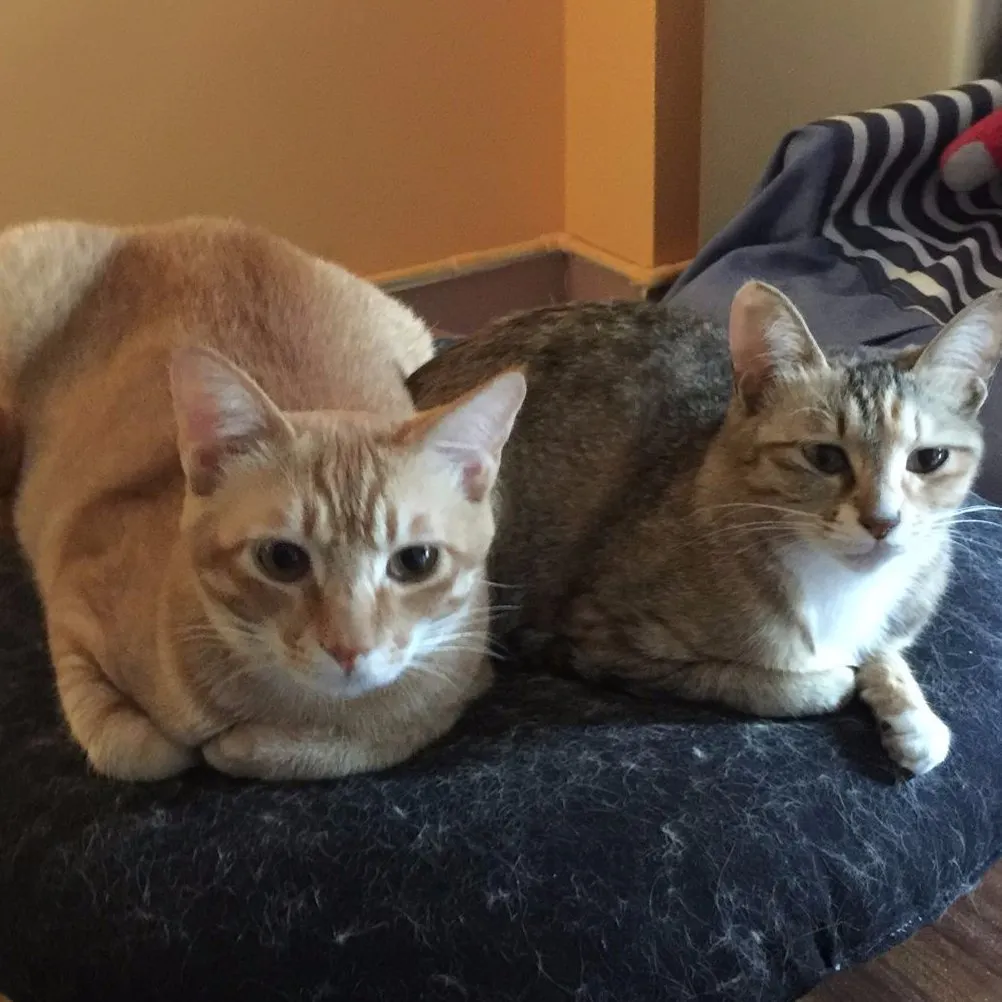# PID Control Line Follower Robot

Cool robot that follows black line. Learn and make a line follower robot with PID controller in 2 hours.

IntermediateFull instructions provided2 hours41,833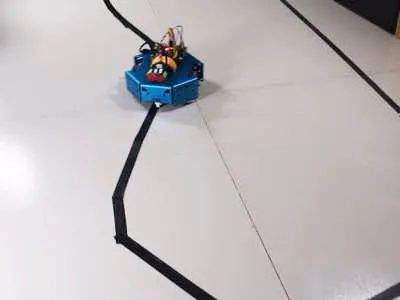## Things used in this project

### Hardware components

 KittenBot Anodized Aluminum Chassis
×1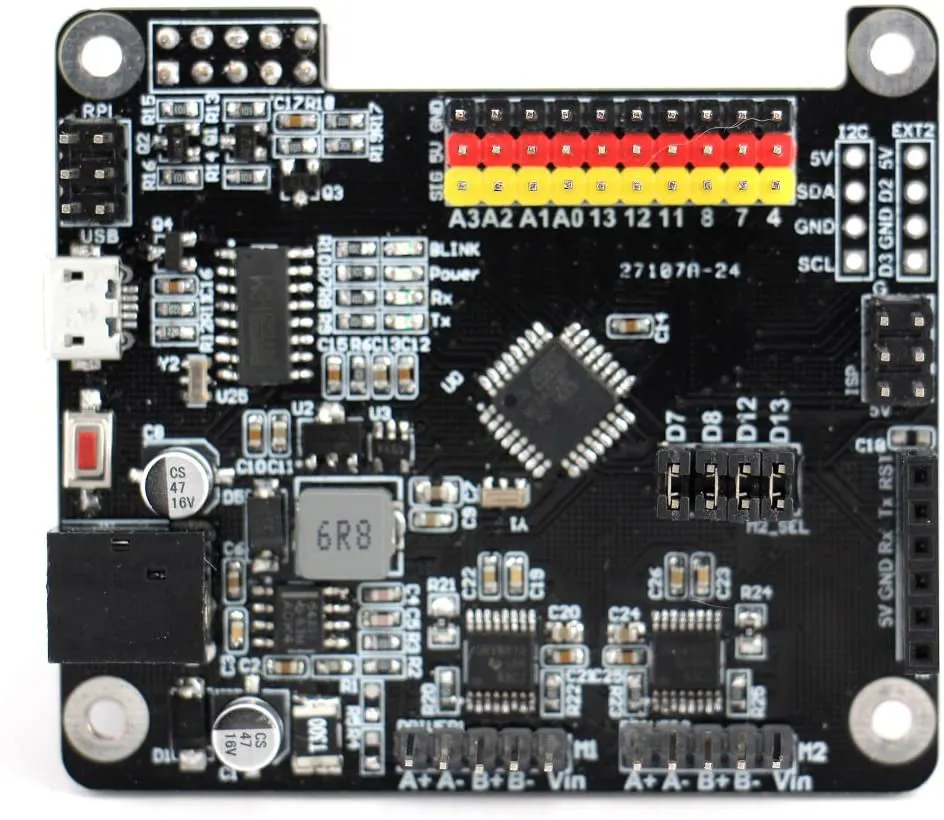KittenBot RosBot Baseboard
×1
 KittenBot Diameter Ball Caster with Metal Ball (Pair)
×1
 KittenBot Rubber Wheel with Motor Hub Set (Pair)
×1
 KittenBot 5-Channel IR Line-follwing Tracker Sensor
×1
 DC motor (generic)
×1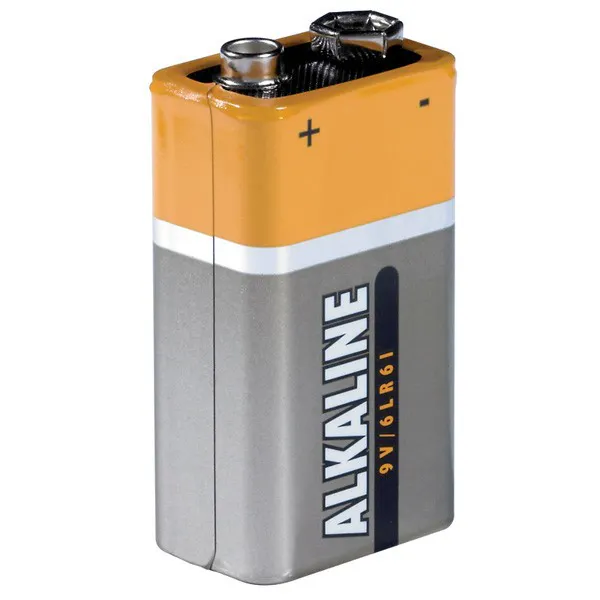9V battery (generic)
×1Arduino UNO
×1

### Software apps and online servicesArduino IDE

## Schematics

### hackster_5yM51Gieht.jpg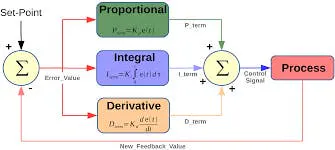## Code

### Line follower robot

Arduino
In the codes, we include a NeoPixel from Adafruit, but that's optional.
```#include <Adafruit_NeoPixel.h>
#define S_NULL 0
#define S_ONTRACE 1

void doDcSpeed(int spdL, int spdR) {
spdR = -spdR;
if (spdL < 0) {
analogWrite(5, 0);
analogWrite(6, -spdL);
} else {
analogWrite(5, spdL);
analogWrite(6, 0);
}

if (spdR < 0) {
analogWrite(9, 0);
analogWrite(10, -spdR);
} else {
analogWrite(9, spdR);
analogWrite(10, 0);
}
}

int bias = 0;
int outlineCnt = 0;

void stateMachine(int a) {
switch (a) {
case B00000:
outlineCnt++;
break;
case B11111:
outlineCnt++;
break;
case B00010:
case B00110:
outlineCnt = 0;
pixels.setPixelColor(2, pixels.Color(0, 50, 0));
bias = 1;
break;
case B00001:
case B00011:
outlineCnt = 0;
pixels.setPixelColor(2, pixels.Color(0, 200, 0));
bias = 2;
break;
case B00100:
outlineCnt = 0;
pixels.setPixelColor(2, pixels.Color(0, 0, 20));
bias = 0;
break;
case B01000:
case B01100:
outlineCnt = 0;
pixels.setPixelColor(2, pixels.Color(50, 0, 0));
bias = -1;
break;
case B10000:
case B11000:
outlineCnt = 0;
pixels.setPixelColor(2, pixels.Color(200, 0, 0));
bias = -2;
break;
default:
Serial.println(a,BIN);
outlineCnt++;
break;
}

pixels.setPixelColor(0, pixels.Color(outlineCnt * 10, 0, 0));
if (outlineCnt > 10) {
doDcSpeed(0,0);
} else {
float ff = 150;
float ctrl = calcPid(bias);
doDcSpeed(ff-ctrl,ff+ctrl);
}
pixels.show();
}

float Kp = 25;
float Ki = 0.15;
float Kd = 1200;
float error, errorLast, erroInte;

float calcPid(float input) {
float errorDiff;
float output;
error = error * 0.7 + input * 0.3; // filter
//error = input;
errorDiff = error - errorLast;
erroInte = constrain(erroInte + error, -50, 50);
output = Kp * error + Ki * erroInte + Kd * errorDiff;
Serial.print(error); Serial.print(' ');
Serial.print(erroInte); Serial.print(' ');
Serial.print(errorDiff); Serial.print(' ');
Serial.println(output);
errorLast = error;

return output;
}

int echoTrace() {
int ret = 0;
int a;
for (int i = 0; i < 5; i++) {
a[i] = constrain((1025 - analogRead(A0 + i)) / 10 - 4, 0, 20);
if (a[i] > 2) ret += (0x1 << i);
}
return ret;
}

void setup() {
Serial.begin(115200);
pixels.begin();
}

int pos;
void loop() {
delay(5);
pos = echoTrace();
stateMachine(pos);

}
```

## Credits

### Christina Zhang

3 projects • 21 followers
Founder of KittenBot

### Rufus Zeng

2 projects • 20 followers
Computer Engineering student at Binghamton University